# tclustICplotGPCM

tclustICplotGPCM plots information criterion as a function of $c_{det}$, $c_{shw}$, $c_{shb}$ and $k$

## Syntax

• tclustICplotGPCM(IC)example
• tclustICplotGPCM(IC,Name,Value)example

## Description

tclustICplotGPCM takes as input the output of function tclustICgpcm (that is a series of matrices which contain the values of the information criteria BIC/ICL/CLA for different values of $k$, $c_{det}$, $c_{shw}$ $c_{shb}$ and type or rotation The plot enables interaction in the sense that, if option databrush has been activated, it is possible to click on a point in the plot and to see the associated classification in the scatter plot matrix.

 tclustICplotGPCM(IC) Plot BIC, ICL and CLA for for Geyser data with all default options.

 tclustICplotGPCM(IC, Name, Value) Example of the use of option datatooltip (all default options).

## Examples

expand all

###Plot BIC, ICL and CLA for for Geyser data with all default options.

Y=load('geyser2.txt');
% Make sure (whenever possible) that units 15, 30 and 69 are inside
% groups which have labels respectively equal to 1, 2 and 3.
UnitsSameGroup=[15 30 69];
nsamp=50;
out=tclustICgpcm(Y,'cleanpool',false,'plots',0,'alpha',0.1,...
'UnitsSameGroup',UnitsSameGroup,'nsamp',nsamp);
tclustICplotGPCM(out);
k=1
k=2
k=3
k=4
k=5
The labels of in the top part of Figure named BIC denote the values of $c_{det}$ ($c_{shw}$) for which IC is minimum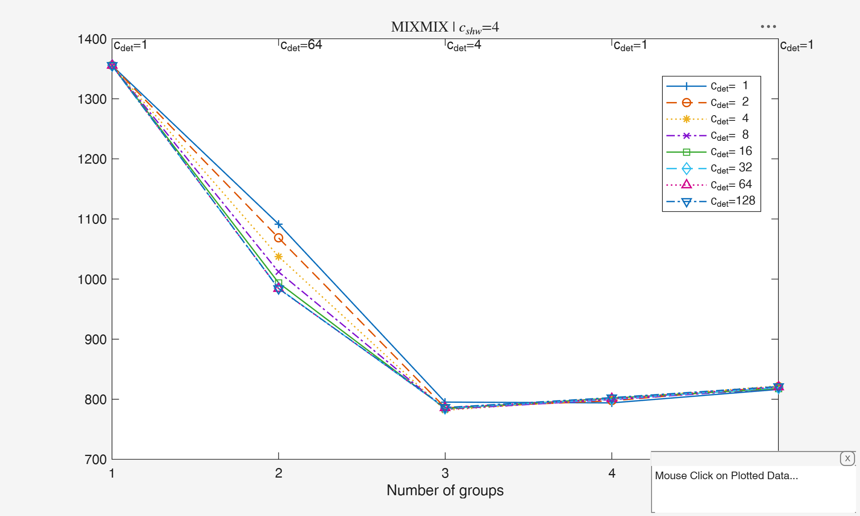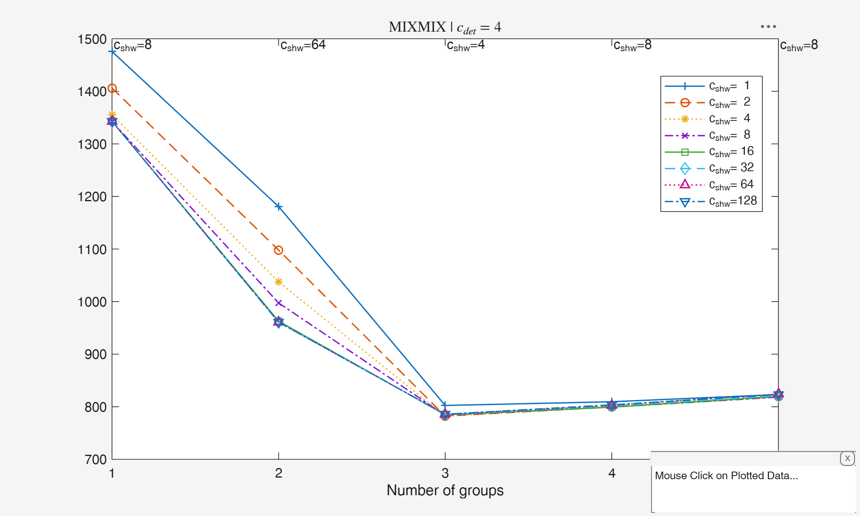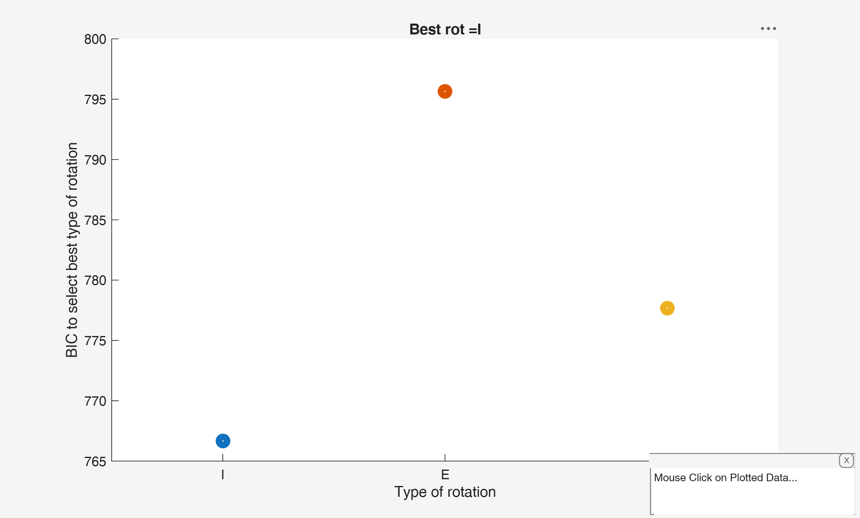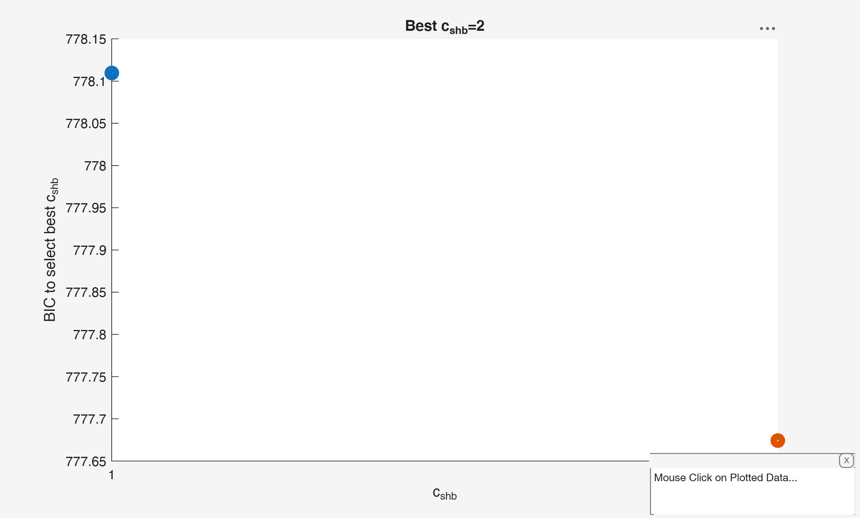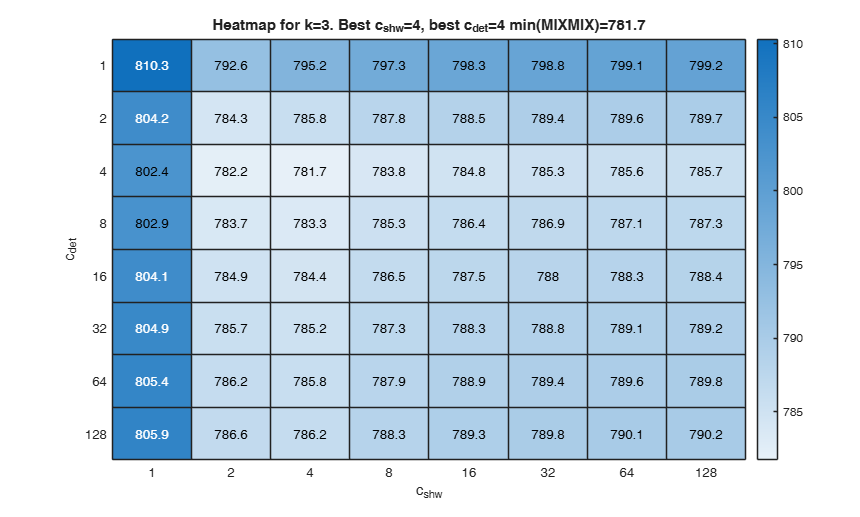### Example of the use of option datatooltip (all default options).

Gives the user the possibility of clicking on the different points and have information about 1) value of k which has been selected 2) value of restriction factor which has been selected 3) values of the information criterion 4) frequency distribution of the associated classification

Y=load('geyser2.txt');
UnitsSameGroup=[15 30 69];
nsamp=50;
out=tclustICgpcm(Y,'cleanpool',false,'plots',0,'alpha',0.1,...
'UnitsSameGroup',UnitsSameGroup,'nsamp',nsamp);
tclustICplotGPCM(out,'datatooltip',1);

## Related Examples

expand all

### Example of the use of option datatooltip (personalized options).

Gives the user the possibility of clicking on the different points and have information about the selected, the step of entry into the subset and the associated label.

datatooltip = struct;
% In this example the style of the datatooltip is 'datatip'. Click on a
% point when the ICplot is displayed.
datatooltip.DisplayStyle = 'datatip';
UnitsSameGroup=[15 30 69];
nsamp=50;
out=tclustICgpcm(Y,'cleanpool',false,'plots',0,'alpha',0.1,...
'UnitsSameGroup',UnitsSameGroup,'nsamp',nsamp);
tclustICplotGPCM(out,'datatooltip',datatooltip);

### Interactive example 1. databrushing from any of the plot which was produced.

% Use all default options for databrush (brush just once)
close all
UnitsSameGroup=[15 30 69];
out=tclustICgpcm(Y,'cleanpool',false,'plots',0,'alpha',0.1,...
'UnitsSameGroup',UnitsSameGroup,'nsamp',nsamp);
tclustICplotGPCM(out,'databrush',1);

### Interactive example 2. Repeated databrushing.

% enable repeated brushing and show boxplots of groups inside diag of spm
databrush=struct;
% Set the shape of the brush
databrush.selectionmode='Rect';
% Enable repeated brushing
databrush.persist='on';
% Include x and y coordinates of brushed solutions inside ICplot
databrush.Label='on';
% Remove x and y coordinated just after btushing
databrush.RemoveLabels='on';
% show boxplots of the groups instead of histograms on the main
% diagonal of the spm
databrush.dispopt='box';
UnitsSameGroup=[15 30 69];
out=tclustICgpcm(Y,'cleanpool',false,'plots',0,'alpha',0.1,...
'UnitsSameGroup',UnitsSameGroup,'nsamp',nsamp);
tclustICplotGPCM(out,'databrush',databrush);

## Input Arguments

### IC — Information criterion to use. Structure.

It contains the following fields.

IC.MIXMIX (or IC.MIXCLA or IC.CLACLA) = 3D array of size length(kk)-times-length(IC.ccdet)-times-length(IC.shw) containinig the value of the penalized classification likelihood.

IC.IDXMIX (or IC.IDXCLA) = cell of size length(kk)-times-length(pa.ccdet)-times-length(pa.ccshw).

Each element of the cell is a vector of length n containinig the assignment of each unit using the classification model.

Value Description
kk

vector containing the values of k (number of components) which have been considered.

ccdet

scalar or vector containing the values of $c_{det}$ (values of the restriction factor for the determinants) which have been considered.

ccshw

scalar or vector containing the values of $c_{shw}$ (values of the restriction factor for the withing groups shape elements) which have been considered.

alpha

scalar value of trimming which has been considered.

BICbest

scalar containing optimal value of BIC (smallest value of BIC).

nameY

cell of length(size(Y,2)) containing the names of the variables of original matrix Y

Data Types: struct

### Name-Value Pair Arguments

Specify optional comma-separated pairs of Name,Value arguments. Name is the argument name and Value is the corresponding value. Name must appear inside single quotes (' '). You can specify several name and value pair arguments in any order as  Name1,Value1,...,NameN,ValueN.

Example:  'tag','myplot' , 'datatooltip','' , 'databrush',1 , 'nameY',{'myY1', 'myY2'} 

### tag —Personalized tag.string.

String which identifies the handle of the plot which is about to be created. The default is to use tag 'pl_ICgpcm'.

Note that if the program finds a plot which has a tag equal to the one specified by the user, then the output of the new plot overwrites the existing one in the same window else a new window is created.

Example:  'tag','myplot' 

Data Types: char

### datatooltip —interactive clicking.empty value (default) | structure.

The default is datatooltip=''.

If datatooltip = 1, the user can select with the mouse a solution in order to have the following information:

1) value of $k$ which has been selected 2) value of $c_{det}$ which has been selected 3) value of $c_{shw}$ which has been selected 4) value of $c_{shb}$ which has been selected 5) values of the information criterion 6) frequency distribution of the associated classification If datatooltip is a structure it may contain the following the fields

Value Description
DisplayStyle

Determines how the data cursor displays. datatip | window.

- datatip displays data cursor information in a small yellow text box attached to a black square marker at a data point you interactively select.

- window displays data cursor information for the data point you interactively select in a floating window within the figure.

SnapToDataVertex

Specifies whether the data cursor snaps to the nearest data value or is located at the actual pointer position. on | off.

- on data cursor snaps to the nearest data value - off data cursor is located at the actual pointer position.

(see the MATLAB function datacursormode or the examples below). Default values are datatooltip.DisplayStyle = 'Window' and datatooltip.SnapToDataVertex = 'on'.

Example:  'datatooltip','' 

Data Types: scalar double or struct

### databrush —interactive mouse brushing.empty value, scalar | structure.

If databrush is an empty value (default), no brushing is done. The activation of this option (databrush is a scalar or a structure) enables the user to select a set of values of IC in the current plot and to see the corresponding classification highlighted in the scatter plot matrix (spm). If spm does not exist it is automatically created. Please, note that the window style of the other figures is set equal to that which contains the IC plot. In other words, if the IC plot is docked all the other figures will be docked too.

DATABRUSH IS A SCALAR. If databrush is a scalar the default selection tool is a rectangular brush and it is possible to brush only once (that is persist='').

DATABRUSH IS A STRUCTURE. If databrush is a structure, it is possible to use all optional arguments of function selectdataFS and the following optional arguments: - databrush.persist = repeated brushing enabled. Persist is an empty value or a scalar containing the strings 'on' or 'off'.

The default value of persist is '', that is brushing is allowed only once.

If persist is 'on' or 'off' brushing can be done as many time as the user requires.

If persist='on' then the unit(s) currently brushed are added to those previously brushed. it is possible, every time a new brushing is done, to use a different color for the brushed solutions.

If persist='off' every time a new brush is performed units previously brushed are removed.

- databrush.Label = add labels (i.e. x=value of k and y=values of IC) of brushed solutions in the ICplot.

Character. [] (default) | '1'.

- dispopt = string which controls how to fill the diagonals in the scatterplot matrix of the brushed solutions. Set dispopt to 'hist' (default) to plot histograms, or 'box' to plot boxplots.

Example:  'databrush',1 

Data Types: single | double | struct

### nameY —variable labels.cell array.

Cell array of strings containing the labels of the variables. As default value, the labels which are added are Y1, ..., Yv.

Example:  'nameY',{'myY1', 'myY2'} 

Data Types: cell